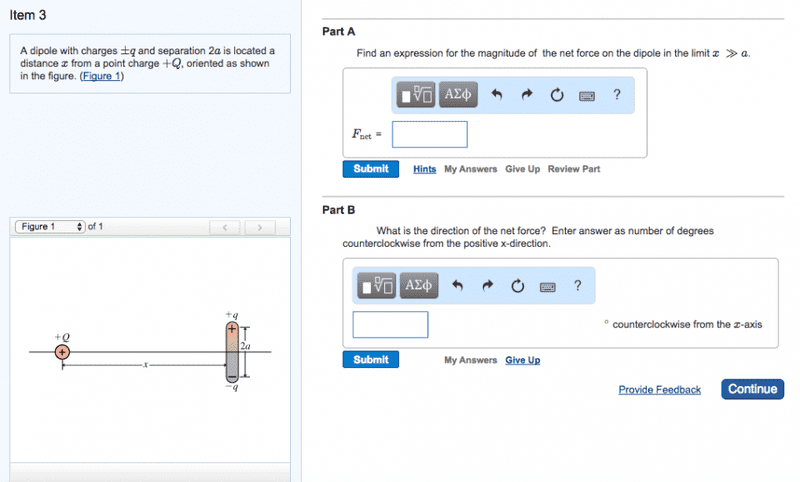# Finding magnitude and direction of net force on a dipole.

## Homework Statement

A dipole with charges ±q and separation 2a is located a distance x from a point charge +Q, oriented as shown in the figure.

Part A:
Find an expression for the magnitude of the net force on the dipole in the limit x≫a.

Part B:
What is the direction of the net force? Enter answer as number of degrees counterclockwise from the positive x-direction.F = kq1q2/r2

## The Attempt at a Solution

I honestly cannot think of a solution for this problem. Currently I am stuck on the dipole piece. How does one come up with an equation using the limit x >> a? I guess a better question would be what that means in terms of figuring out the net Force on the dipole. I am simply confused on where to start or how to begin besides using F = kq1q2/r2

haruspex
Homework Helper
Gold Member
2020 Award
F = kq1q2/r2
Mostly, students start with an equation they have learnt specifically related to dipoles. I cannot tell whether you are expected to start from there or from the equation you quoted. Either will do, but it is a bit more work using the latter. Since that's the equation you quote, I'll assume for now that is the method to use.

If you were asked to find the force the dipole exerts on the point charge, could you do that?

Mostly, students start with an equation they have learnt specifically related to dipoles. I cannot tell whether you are expected to start from there or from the equation you quoted. Either will do, but it is a bit more work using the latter. Since that's the equation you quote, I'll assume for now that is the method to use.

If you were asked to find the force the dipole exerts on the point charge, could you do that?
Well the dipole exerts both a positive and negative force onto the +Q charge which would simply cancel out correct? Since they are equidistant thus equal, but opposite magnitudes.

haruspex